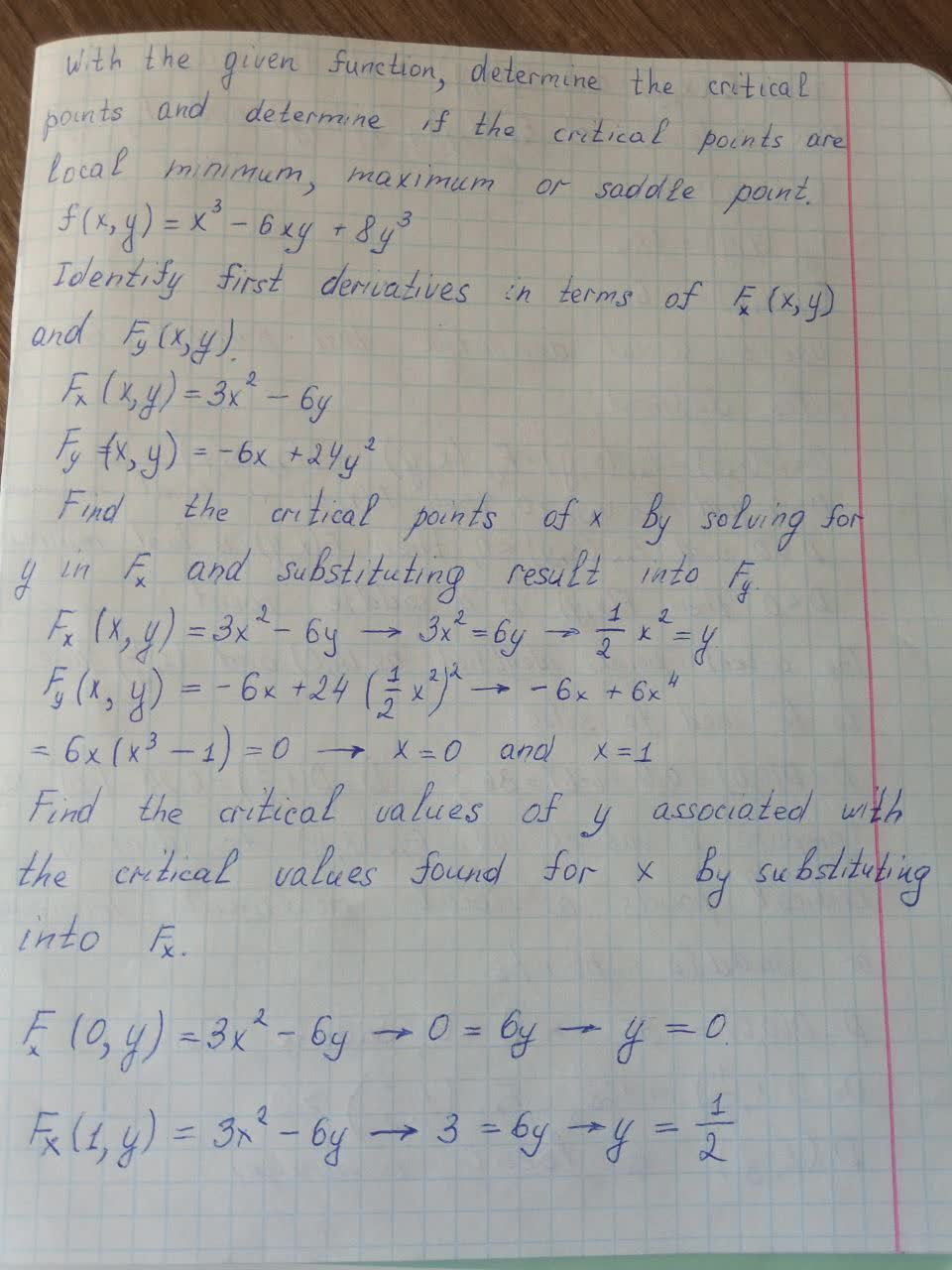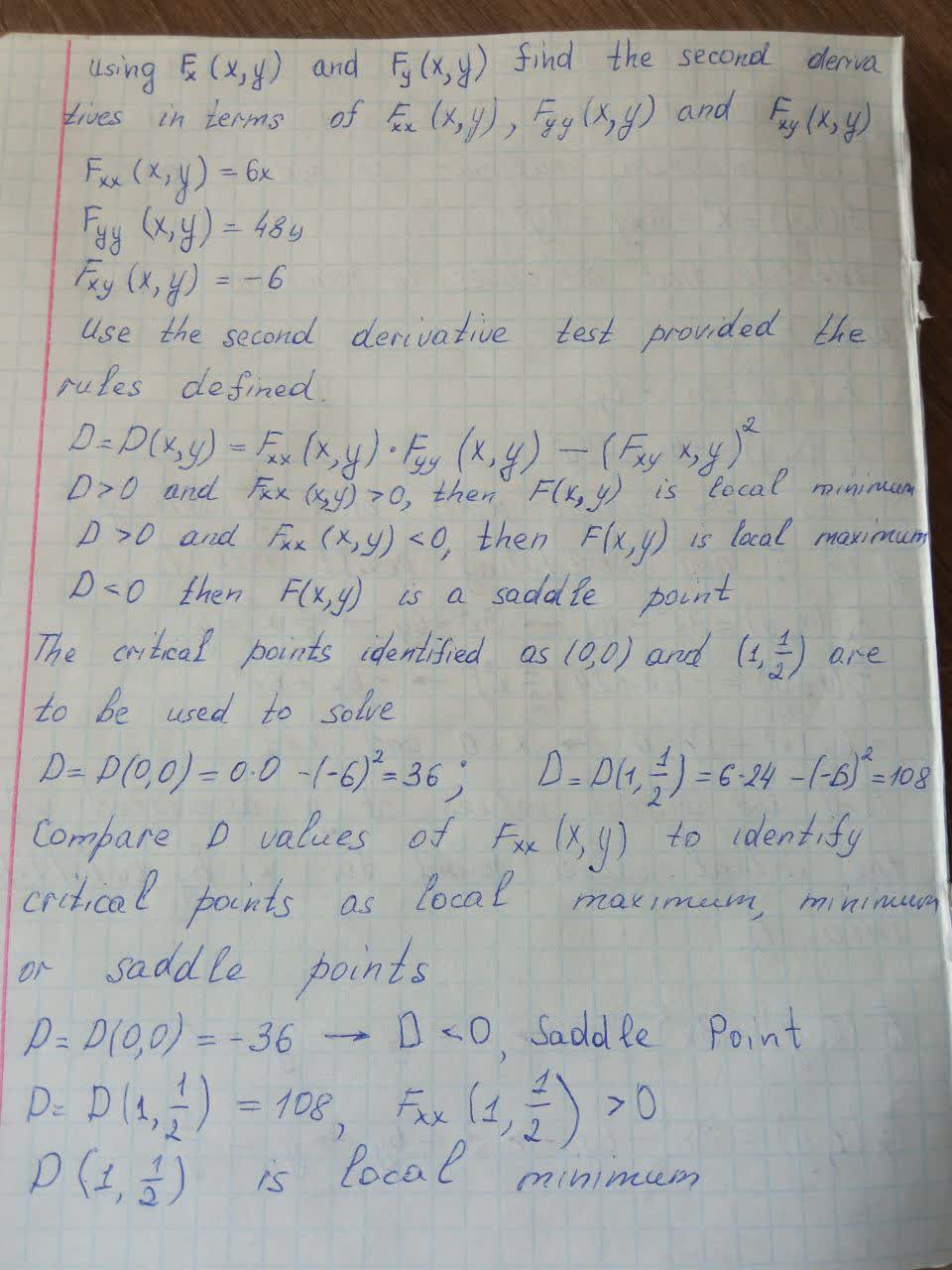Question# Find the local maximum and minimum values and saddle points of the function. If you have three-dimensional graphing software, graph the function with a domain and viewpoint that reveal all the important aspects of the function. f(x,y)=x^3-6xy+8y^3

Analyzing functions
ANSWEREDFind the local maximum and minimum values and saddle points of the function. If you have three-dimensional graphing software, graph the function with a domain and viewpoint that reveal all the important aspects of the function.
$$f(x,y)=x^3-6xy+8y^3$$2021-05-152021-09-29

With the given function, determine the critical points and determine if the critical points are local minimum, maximum or saddle point.

$$f(x,y)=x^3-6xy+8y^3$$

Identify first derivatives in terms of $$F_x(x,y)$$ and $$F_y(x,y)$$

$$F_x(x,y)=3x^2-6y$$

$$F_y(x,y)=-6x+24y^2$$

Find the critical points of x by solving for y in $$F_x$$ and substituting result into $$F_y$$

$$F_x(x,y)=3x^2-6y\to3x^2=6y\to\frac{1}{2}x^2=y$$

$$F_y(x,y)=-6x+24(\frac{1}{2}x^2)^2\to-6x+6x^4$$

$$=6x(x^3-1)=0\to x=0$$ and $$x=1$$

Find the critical values of y associated with the critical values found for x by substituting into $$F_x$$

$$F_x(0,y)=3x^2-6y\to0=6y\to y=0$$

$$F_x(1,y)=3x^2-6y\to3=6y\to y=\frac{1}{2}$$

using $$F_x(x,y)$$ and $$F_y(x,y)$$ find the second derivatives in terms of $$F_{xx}(x,y),\ F_{yy}(x,y)$$ and $$F_{xy}(x,y)$$

$$F_{xx}(x,y)=6x$$

$$F_{yy}(x,y)=48y$$

$$F_{xy}=-6$$

Use the second derivative test provided the rules defined.

$$D=D(x,y)=F_{xx}(x,y)\cdot F_{yy}(x,y)-(F_{xy}x,y)^2$$

$$D>0$$ and $$F_{xx}(x,y)<0$$, then $$F(x,y)$$ is local maximum $$D<0$$ then $$F(x,y)$$ is a saddle point

The critical points identified as (0,0) and $$(1,\frac{1}{2})$$ are to be used to solve

$$D=D(0,0)=0\cdot0-(-6)^2=36;\ D=D(1,\frac{1}{2})=6\cdot24-(-6)^2=108$$

Compare D values of $$F_{xx}(x,y)$$ to indentify critical points as local maximum, minimum or saddle points

$$D=D(0,0)=-36\to D<0$$ Saddle point

$$D=D(1,\frac{1}{2})=108,\ F_{xx}(1,\frac{1}{2})>0$$

$$D(1,\frac{1}{2})$$ is local minimum